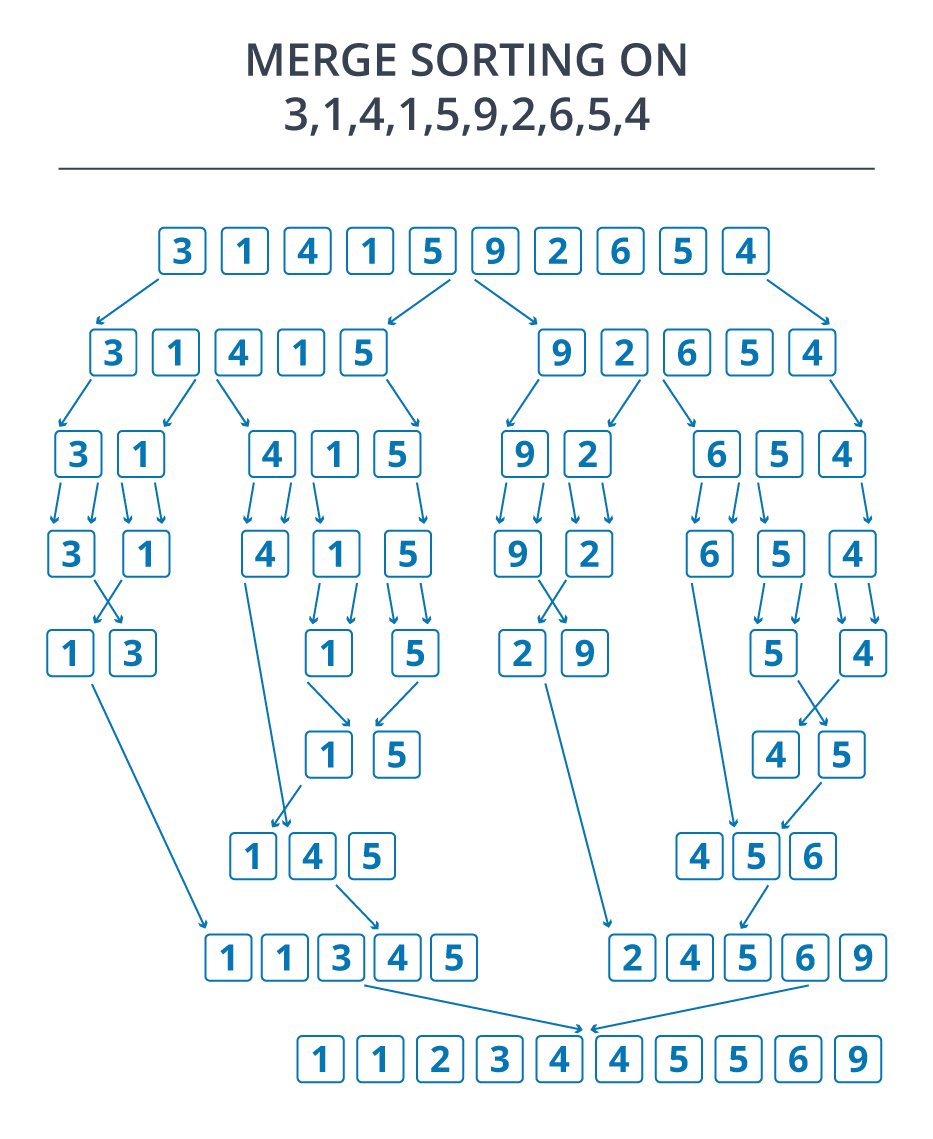# How to implement Merge Sort in Python?10 / 11 Blog from Python Programs

This blog is based on the divide and conquer approach. Merge Sort is a “divide and conquer” algorithm where the problem is divided into subproblems and is then merged to conquer the solution. This blog on Merge Sort in Python will walk you through the below topics in detail –

## What is Merge Sort in Python?

Merge Sort is based on the divide and conquer algorithm where the input array is divided into two halves, then sorted separately and merged back to reach the solution. The function merge() is used for merging the sorted arrays.

## The Divide and Conquer approach

• The array is split in half and the process is repeated with each half until each half is of size 1 or 0.
• The array of size 1 is trivially sorted.
• Now the two sorted arrays are combined into one big array. And this is continued until all the elements are combined and the array is sorted.

Here is a visualization of merge sort to clear the picture for you

Input Array = [3,1,4,1,5,9,2,6,5,4]Now, let us move on to the implementation.

## Implementing Merge Sort in Python

```def mergeSort(nlist):
print("Splitting ",nlist)
if len(nlist)>1:
mid = len(nlist)//2
lefthalf = nlist[:mid]
righthalf = nlist[mid:]

mergeSort(lefthalf)
mergeSort(righthalf)
i=j=k=0
while i < len(lefthalf) and j < len(righthalf):
if lefthalf[i] < righthalf[j]:
nlist[k]=lefthalf[i]
i=i+1
else:
nlist[k]=righthalf[j]
j=j+1
k=k+1

while i < len(lefthalf):
nlist[k]=lefthalf[i]
i=i+1
k=k+1

while j < len(righthalf):
nlist[k]=righthalf[j]
j=j+1
k=k+1
print("Merging ",nlist)

nlist = [3,1,4,1,5,9,2,6,5,4]
mergeSort(nlist)
print(nlist)
```

Output:

\$python main.py
(‘Splitting ‘, [3, 1, 4, 1, 5, 9, 2, 6, 5, 4])
(‘Splitting ‘, [3, 1, 4, 1, 5])
(‘Splitting ‘, [3, 1])
(‘Splitting ‘, )
(‘Merging ‘, )
(‘Splitting ‘, )
(‘Merging ‘, )
(‘Merging ‘, [1, 3])
(‘Splitting ‘, [4, 1, 5])
(‘Splitting ‘, )
(‘Merging ‘, )
(‘Splitting ‘, [1, 5])
(‘Splitting ‘, )
(‘Merging ‘, )
(‘Splitting ‘, )
(‘Merging ‘, )
(‘Merging ‘, [1, 5])
(‘Merging ‘, [1, 4, 5])
(‘Merging ‘, [1, 1, 3, 4, 5])
(‘Splitting ‘, [9, 2, 6, 5, 4])
(‘Splitting ‘, [9, 2])
(‘Splitting ‘, )
(‘Merging ‘, )
(‘Splitting ‘, )
(‘Merging ‘, )
(‘Merging ‘, [2, 9])
(‘Splitting ‘, [6, 5, 4])
(‘Splitting ‘, )
(‘Merging ‘, )
(‘Splitting ‘, [5, 4])
(‘Splitting ‘, )
(‘Merging ‘, )
(‘Splitting ‘, )
(‘Merging ‘, )
(‘Merging ‘, [4, 5])
(‘Merging ‘, [4, 5, 6])
(‘Merging ‘, [2, 4, 5, 6, 9])
(‘Merging ‘, [1, 1, 2, 3, 4, 4, 5, 5, 6, 9])
[1, 1, 2, 3, 4, 4, 5, 5, 6, 9]

Flow Chart for the implementation of Merge Sort## Advantages and Usage of Merge Sort

Most of the other algorithms perform bad with sequential data structures like files and linked lists. In these structures accessing a random element takes linear time, not regular constant time. And the nature of the merge sort makes it easy and fast for such data structures. One of the best features of merge sort is its low number of compares. It makes O(n * log(n)) number of compares, but the constant factor is good as compared to quicksort, which makes it useful when the compare function is a slow operation. Also, the divide-and-conquer approach of merge sort makes it convenient for parallel processing.

With this, we come to an end of this blog on “How to implement Merge Sort in Python”. I hope the content added some value to your knowledge in Python. To get in-depth knowledge on Python along with its various applications, you can enroll for live Python Certification Training with 24/7 support and lifetime access.

Upcoming Batches For Data Science with Python Certification Course
Course NameDate
Data Science with Python Certification Course

Class Starts on 10th June,2023

10th June

SAT&SUN (Weekend Batch)
View Details
Data Science with Python Certification Course

Class Starts on 5th August,2023

5th August

SAT&SUN (Weekend Batch)
View DetailsREGISTER FOR FREE WEBINARThank you for registering Join Edureka Meetup community for 100+ Free Webinars each month